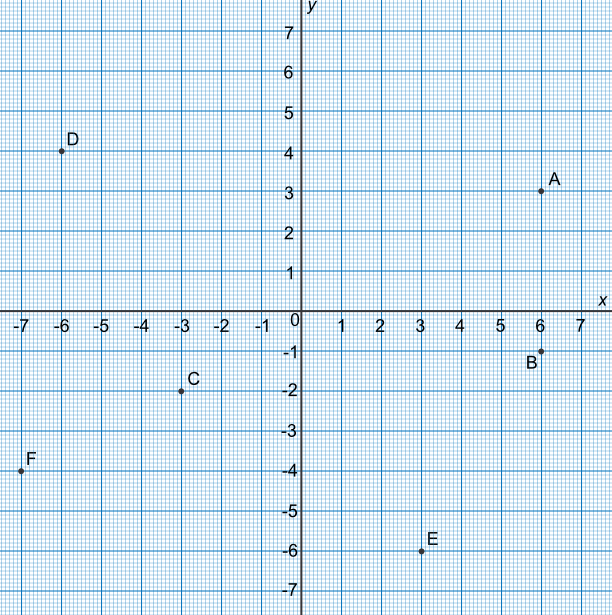Points can also be plotted using negative numbers. The x-axis can be extended leftwards from zero (decreasing in value) and the y-axis extended downwards (also decreasing in value).

The point at which the x-axis and the y-axis cross is known as the origin.

Point A on the grid, below, is at +6 along and +3 up and the coordinates are given as (6, 3). Point B is also 6 along the x-axis, but is at -1 on the y-axis, and is therefore at (6, -1). Any point in this quadrant of the graph (between the two axis lines) will have a positive x value and a negative y value.

Point C is at -3 along the x axis, and at -2 down the y axis. The coordinate of C is (-3, -2). All points between the axes in this quadrant of the graph will have both a negative x and a negative y value

Finally, point D is -6 along and +4 up, so the coordinate of the point is (-6, 4).## Example 1

What is the coordinate of point E?

Three along the x-axis; then 6 down the y-axis.